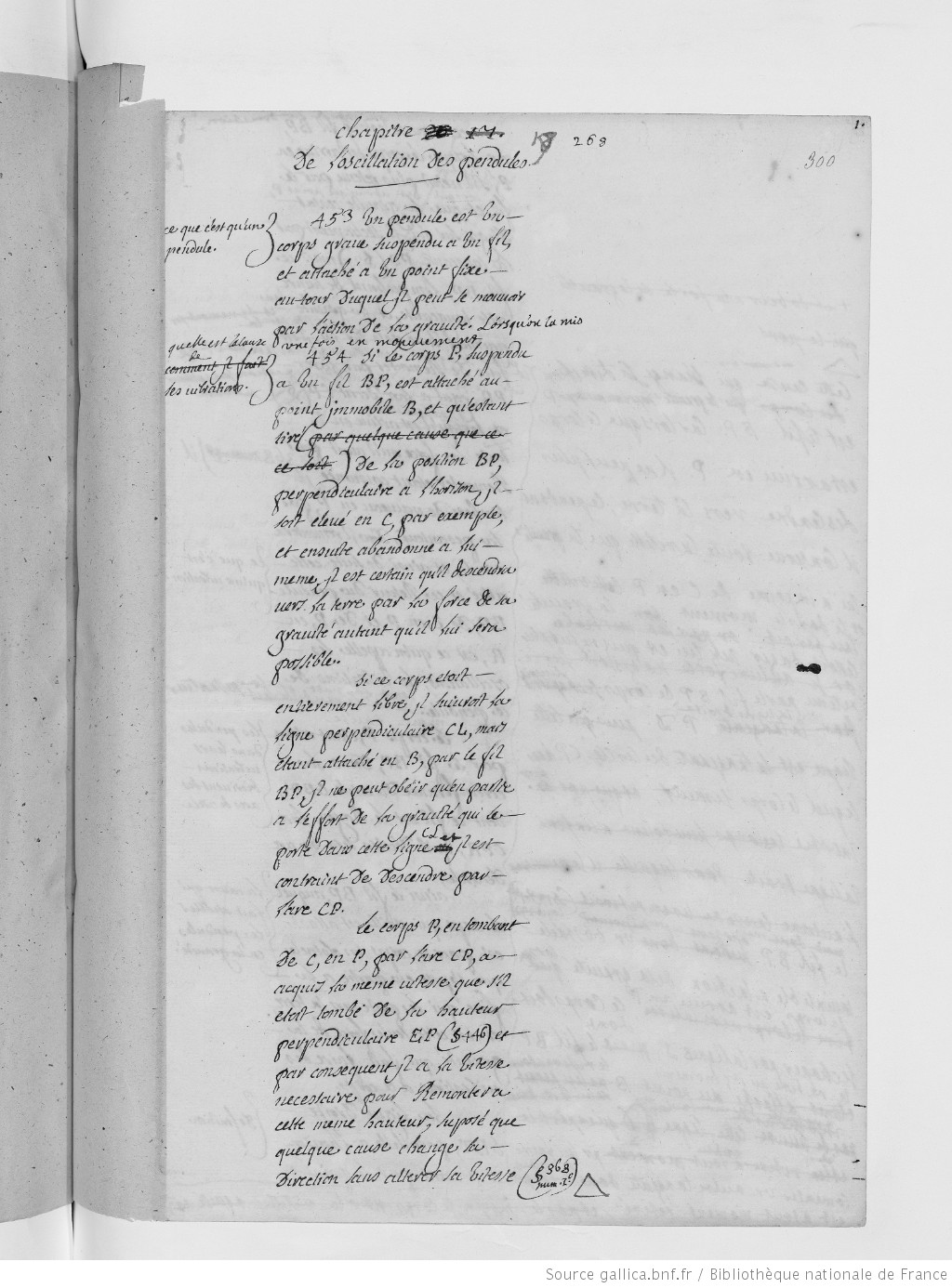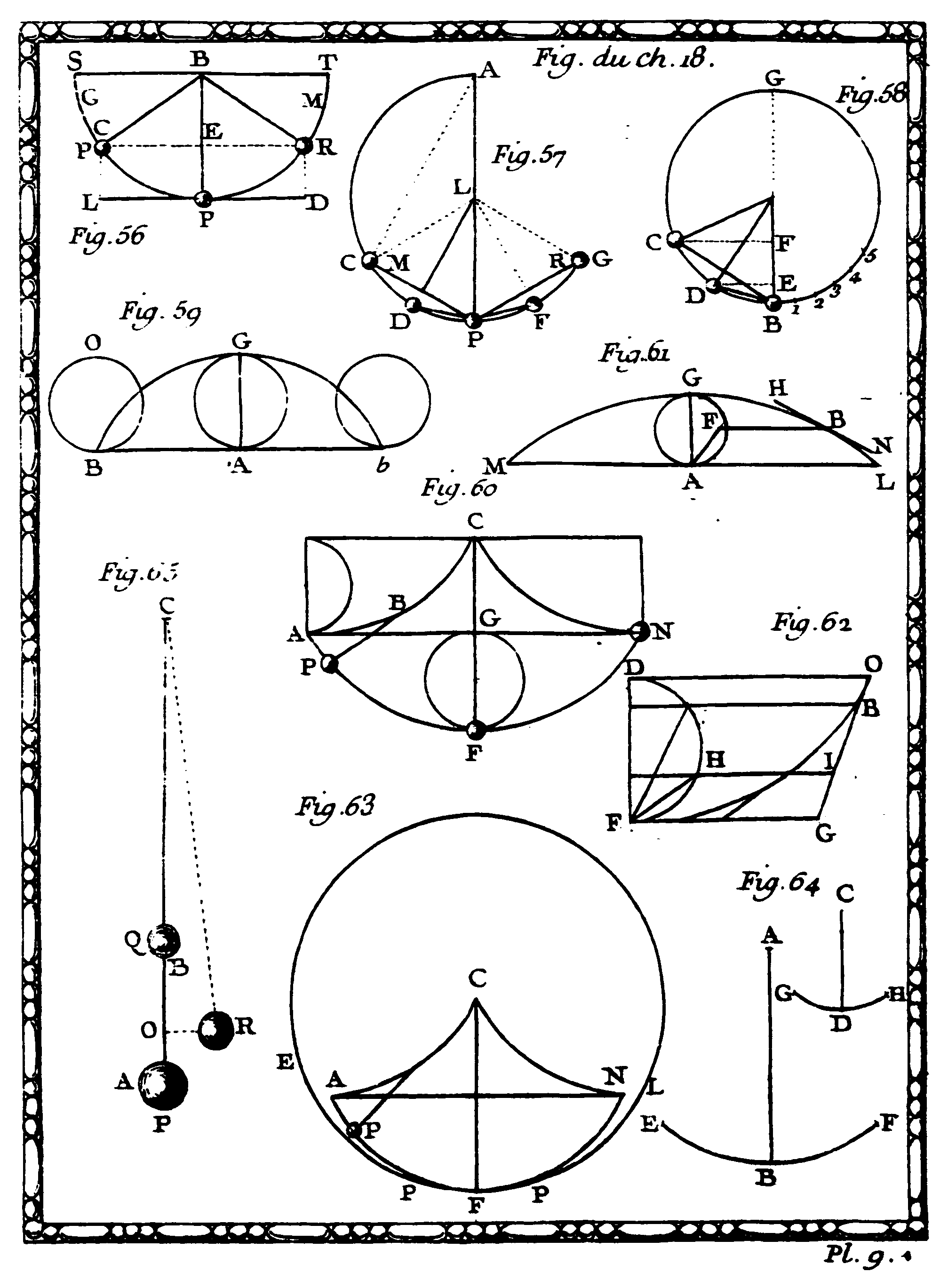Chapter 18. Of the Oscillation of PendulumsThis chapter explores how gravitational forces act on objects by exploring the motion of pendulums. Du Châtelet defines “pendulum” as follows (InstPhy, § 443):

A pendulum is a heavy body hanging from a thread and attached to a fixed point. Once set in motion, it can move around the fixed point by the action of gravity. (my own translation)

In Fig. 56 it is explained how a pendulum works: Since the body is fixed with the thread BP in point P, the body follows the arc CP. When the body P is drawn upwards, the force of gravity accelerates it back to the original position. Then the body swings in the opposite direction to a height equal to the original position (due to the law of inertia.) The back and forth movement of the pendulum BP, from C to P, and from P to R is called the oscillations of the pendulum.A simple pendulum is an idealization in which the whole mass is considered as concentrated at a point (InstPhy, § 447). A mass point is suspended from a massless, rigid thread and can therefore only move in two dimensions on a circular path around the suspension. Its only degree of freedom is the deflection around an equilibrium position. In reality (i.e., in experimental practise) air resistance and friction slows down the swing. If there were no friction to interfere and if all resistances to motion were removed,a pendulum set in motion would swing back and forth in a straight line and would never come to rest (InstPhy, §§ 450-451).

Du Châtelet honors Galileo who was the first to examine pendulums (InstPhys, § 455):

Galileo was the first who imagined a heavy suspended body on a wire, & measured time in Astronomical observations and in physical experiments, by vibrations: thus, he can be considered as the inventor of pendulum clocks. (my own translation)

Galileo found out that periods of smaller angles were constant and assumed that this was correct for every angle, an assumption which we now know to be wrong. In light of this conclusion, Galileo used the pendulum in order to measure short time periods. Following Galileo’s epochal analysis of pendulum motion, Huygens provided the first accurate measure of time. Huygens realized that for practical purposes regarding timekeeping devices it is advisable that one keeps the arc of the swing of a long pendulum relatively small. In the case of small swings of a few degrees, the pendulum approximates isochronousness. That means: With the assumption of small angles, the frequency and period of the pendulum are independent of the initial angular displacement amplitude. (The time for one complete cycle, a left swing and a right swing, is called the period.  The frequency is the number of back and forth swings in a certain length of time.) Huygens’ discovery of the isochronous nature of cycloidal motion rested upon the new geometrical analysis of the cycloid curve. Du Châtelet gives a detailed geometrical description and explanation of Huygens’ method. She refers to Huygens’ Horologium Oscillatorium (1673) and to John Wallis’ Tractatus Duo (1659). In both works Christopher Wren’s solution of the problems on the cycloid is discussed, which had been proposed by Blaise Pascal (InstPhy, § 466; Fig. 60):

A cycloid is the curve traced by a point on the rim of a circular wheel as the wheel rolls along a straight line without slipping. This is the curve of fastest descent under constant gravity (In §§ 469-470 Du Châtelet shortly discusses a paradoxical property of the cycloid, known as the wheel paradox. This passage is missing in the Amsterdam edition of 1742. ) Huygens had failed to realize that the cycloid also represented the “least time” path of descent of a body in a vertical plane. Johann I Bernoulli solved this problem (InstPhy, § 469):

The famous Маthématicien Mr. Jean Bernoulli, who had proposed the problem of the line with the fastest descent, solved it by means of the dioptric. He demonstrated that any ray broken in the atmosphere must describe a cycloid. This Geometer assumed that light, by crossing heterogeneous density media, must be transmitted by the shortest time path, as Fermat had claimed against Descartes, and as Mr Huyghens and Mr Leibnits had confirmed it in favor for Fermat. (my own translation)

Johann Bernoulli’s solution was originally published in the Acta Eruditorum in May 1697 (pp. 206-211). This solution is based on the analogy to Pierre de Fermat’s optical principle of least time. Fermat’s least-time principle is equivalent to the optical law of refraction: a light ray travels between two points in a vertical plane in a medium of continuously varying index of refraction. Bernoulli realized that the solution of the problem of the least-time of fall between two points (the brachistochrone) was the same as the cycloid of Huygens (the tautochrone). Several important mathematicians responded to Bernoulli’s approach, which became a powerful method to solve minimum and maximum problems, later called variational calculus.

Du Châtelet remarks that Leibniz, in §22 of the Discours de métaphysique (1686), interpreted Fermat’s principle as an example for the principle of sufficient reason (InstPhy, § 472):

It is comprehensible with what pleasure Mr. de Leibnits adopted an opinion that has its origin in the principle of the sufficient reason; Fermât claimed that the ray of light follows neither a straight line nor the shortest way, but travels in the shortest possible time, since otherwise there would be no reason for the path of the ray of light when it is refracted. (my own translation)

Du Châtelet also mentions Jean Jacques d’Ortous de Mairan’s discussion of Fermat’s principle (InstPhy, § 476) in the Mémoires de l’Académie royale des sciences (“Sur la reflexion des corps,” 1722, pp. 102-119), referring to her own work on optics (InstPhy, § 471). From Fermat’s principle, one can derive the law of reflection (known as Snell-Descartes law) which describes the relationship between the angles of incidence and refraction, when referring to light or other waves passing through a boundary between two different isotropic media, such as water, glass, or air.

Since the force of gravity is greater near the poles and less at equator, a pendulum will swing slowest when closest to the equator. This fact was confirmed by Jean Richer’s voyage to Cayenne in 1672-73, which destroyed Huygens’ vision of a universal standard of length (thus, time). In his Observations astronomique et physiques faites en l’Isle de Caienne published in Paris in 1679, Richer reported that measurements agreed on a length shorter by 1-3/4 lignes than that found for Paris (see InstPhy, § 477-479). On reading Richer’s report Huygens reasoned that the earth’s rotation produced a centrifugal force that diminished the weights of bodies by a factor dependent on their latitude. A body at either pole suffered no diminution; at the equator it underwent a maximum decreased of roughly of its weight. Translated into horological terms, the diminution of weight by centrifugal force meant that a clock regulated to mean time at the pole would fall behind at the equator by a bit more than 2-1/2 minutes a day. Faced with the fact of geographical variations in the length of a pendulum, Huygens turned to his mechanical theory of weight and to the measure of the perturbing centrifugal force. Newton used the data presented in the report by Jean Richer of his 1672 visit to Cayenne, that of Edmond Halley to St. Helena in 1677, and that of Varin and Des Hayes’ voyage to Martinique and Guadeloupe in 1682 for his calculations in the third book of the Principia (see InstPhy, § 482).

In line with Huygens Du Châtelet distinguishes a simple pendulum from a compound pendulum. A compound pendulum will oscillate like a simple pendulum, but with some restrictions regarding the axis of oscillation and the axis of gravity (InstPhy, § 485). Huygens based the determination of the center of oscillation of a compound pendulum on a principle, named variously after Torricelli and Mersenne: When the center of gravity of a system falls from rest and then rises again to rest, it begins and ends at the same height, irrespective of the changes of relative position within the system itself. By means of this principle Huygens was able to derive a simple pendulum that was isochronous to a compound pendulum. As will be shown in the next chapters, Huygens analysis delivered a breakthrough: it helped to clarify an important physical quantity, to be called “vis viva,” i.e., the capacity of a body to do work.

******************

Compae the entry PENDULE, s. m. (Méchanique.) in d’Alembert’s and Diderot’s Encyclopédie large passages are taken verbatim from Du Châtelet’s Chapter 13. See also the entry “Pendule” in: Johann Heinrich Samuel Formey (Hg.): Dictionnaire instructif, où l’on trouve les principaux termes des sciences et des arts dont l’explication peut être utile ou agréable aux personnes qui n’ont pas fait des études approfondies. Halle: Gebauer.

##### Back to main project | Next chapter